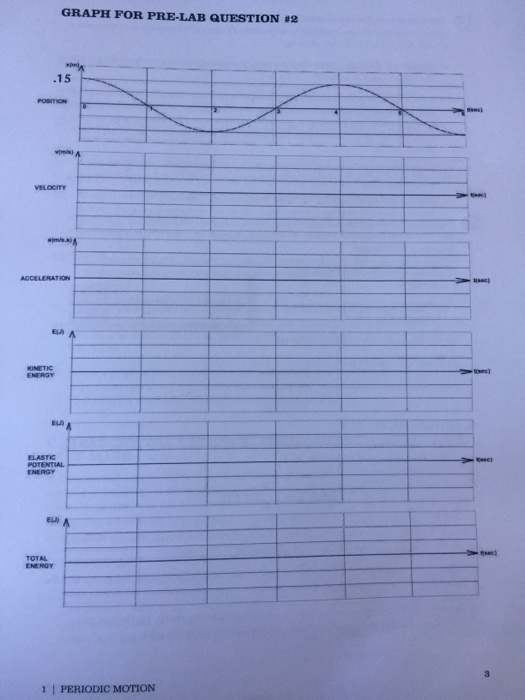# Problem: The graph on the next page shows the displacement of the block as a function of time (this is the same mass-spring situation as problem 1 but with a different mass). The maximum displacement is 15 cm. (The graph for this exercise is provided at the end of this lab on page 7. For your records, trace your solutions from your completed graphs into your lab notebook, copy your solution, and fasten the copy into your notebook.) a) If k = 150 N/m, calculate the mass of the block. b) Draw the graphs for velocity and acceleration of the motion. On the graphs, label the maximum values of velocity and acceleration, respectively. c) Draw the graph for kinetic energy and calculate and label on the graph the maximum value of KEd) Draw the graph for the elastic potential energy and calculate and label on the graph the maximum value of the elastic PE.e) Calculate the numerical value and draw the graph for the total energy.

###### FREE Expert Solution

a)

$\overline{){\mathbit{T}}{\mathbf{=}}{\mathbf{2}}{\mathbit{\pi }}\sqrt{\frac{\mathbf{m}}{\mathbf{k}}}}$

Period of oscillation, T = 4s.

k = 150 N/m

We'll solve for m and calculate:

92% (338 ratings)###### Problem Details

The graph on the next page shows the displacement of the block as a function of time (this is the same mass-spring situation as problem 1 but with a different mass). The maximum displacement is 15 cm. (The graph for this exercise is provided at the end of this lab on page 7. For your records, trace your solutions from your completed graphs into your lab notebook, copy your solution, and fasten the copy into your notebook.)

a) If k = 150 N/m, calculate the mass of the block.

b) Draw the graphs for velocity and acceleration of the motion. On the graphs, label the maximum values of velocity and acceleration, respectively.

c) Draw the graph for kinetic energy and calculate and label on the graph the maximum value of KE

d) Draw the graph for the elastic potential energy and calculate and label on the graph the maximum value of the elastic PE.

e) Calculate the numerical value and draw the graph for the total energy.